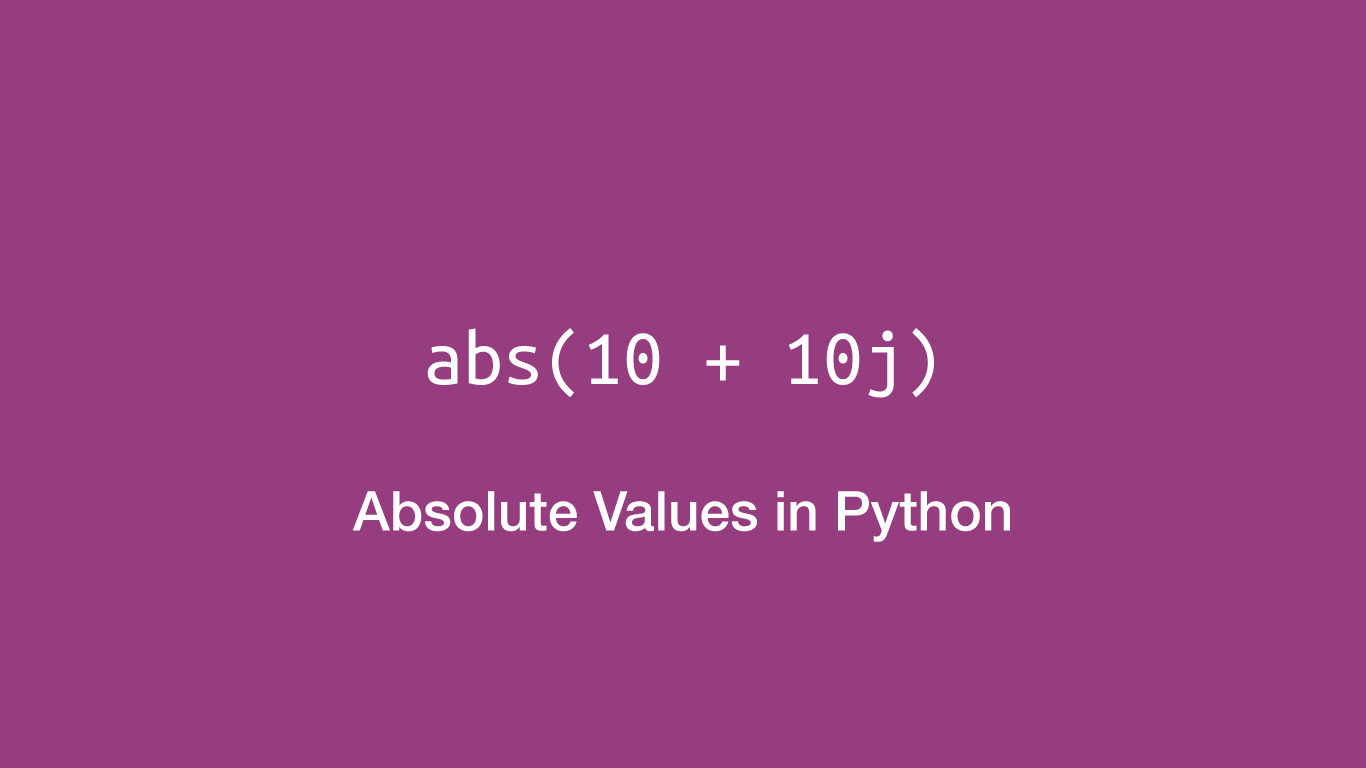# How to get the Absolute Value of a Number in PythonTo get the absolute value of a number in Python, use the `abs()` method. This method will convert integers and floating-point numbers to their absolute equivalents and for complex numbers their magnitude.

## Get the Absolute Value of a Number

To get the absolute value of a floating-point or integer pass it inside the `()` (parenthesis) of the `abs()` method. Let's try out a couple of examples and print the results.

``````decimal = -1.354
integer = -34564

dec_abs = abs(decimal)
int_abs = abs(integer)

print(dec_abs)
print(int_abs)
``````
``````1.354
34564
``````

## Get the Absolute Value of a Complex Number

A complex number consists of a real number and an imaginary unit to represent a number that can't be represented numerically. If we pass a complex number into `abs()` we will get its magnitude.

``````comp = 10 + 10j

comp_abs = abs(comp)

print(comp_abs)
``````
``````14.142135623730951
``````

## Conclusion

You now know how to get the absolute value of any given number using `abs()` in Python

#### Related Tutorials### How to Calculate Mean, Median, Mode and Range in Python

October 07, 2020### How to Create and Use Lists (Arrays) in Python

September 01, 2020### How to Create Django Template Absolute URL (Dynamic URL Generation)

July 27, 2021### Find Maximum Value in a List in Python

September 22, 2021### How to use Sets in Python

September 15, 2020### How to Use Argparse in Python

September 25, 2020